# Laboratorium Zimnych Atomów przy Powierzchni

Laboratory of Cold Atoms Near Surfaces

## Evanescent wave properties

Penetration depth

We define the penetration depth d reflecting the decrease of the evanescent wave amplitude in the "z" direction: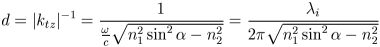(8)Fig. 2. Penetration depth as a function of the incident angle λ = 794.5 nm, n1 = 1.45, n2 = 1, αc = 43.60

The d is a decreasing function of the angle of incidence and for α-αo~0,1° "d" is of the order of a wavelength.

Poynting vector

In spite of the existence of the electric field in the less dense medium, the mean energy flux along the "z" direction equals 0. Let us take the Poynting vector: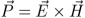(9)

For the e.g. TE polarization of the incident beam we get: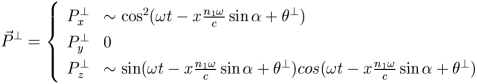(10)

The average flux through the boundary over a period is 0.

Goos-Hänchen shift 

Let us consider the laterally limited beam totally reflected at the interface of two dielectrics. It turns out that in this case the points of incidence and reflection are spatially shifted: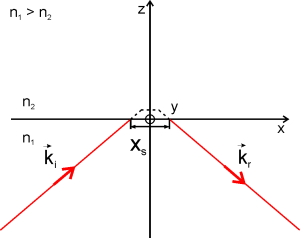Fig. 3. Goos-Hänchen effect Dashed line shows in a very symbolic way the direction of propagation of the evanescent wave.

The magnitude of the Goos-Hänchen effect depends on the polarization of the incident beam: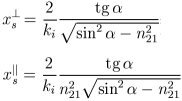(11)

Exemplary reference

 F. de Fornel, Evanescent Waves, Springer, Berlin (2000)
 F. Goos, H. Hänchen, Ann. Phys. 1, 333 (1947)